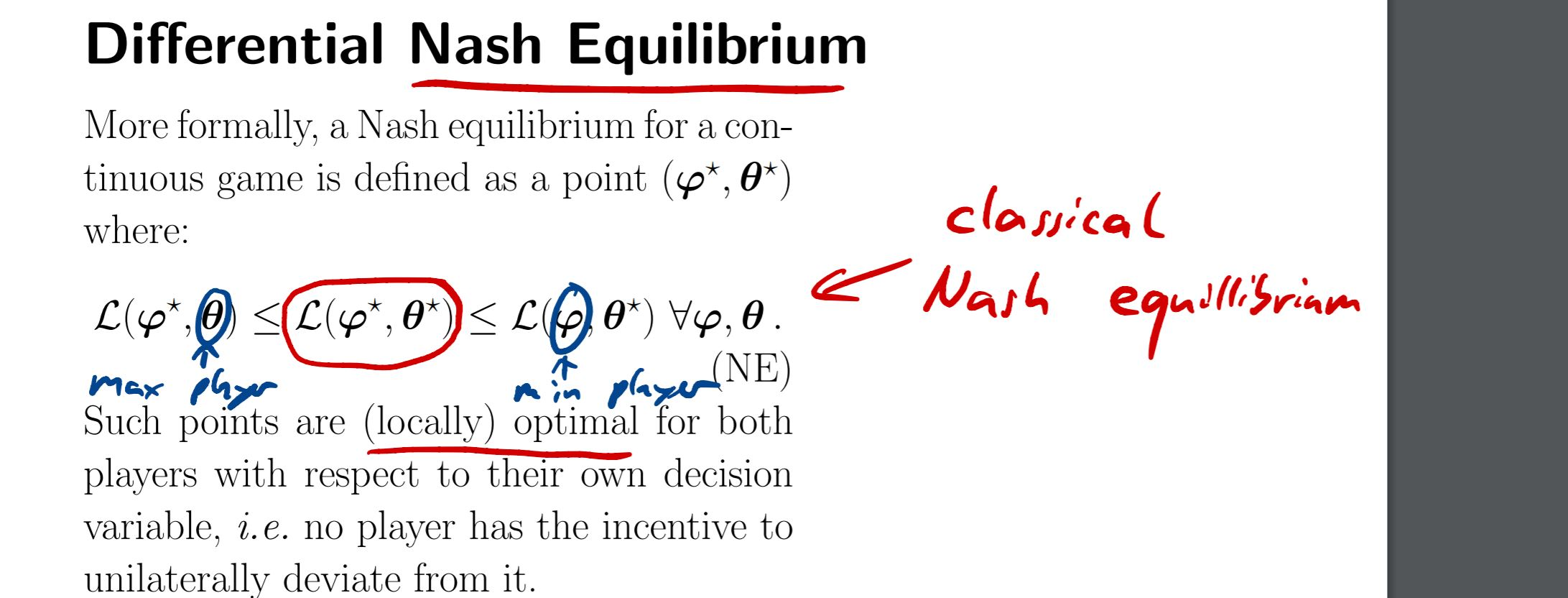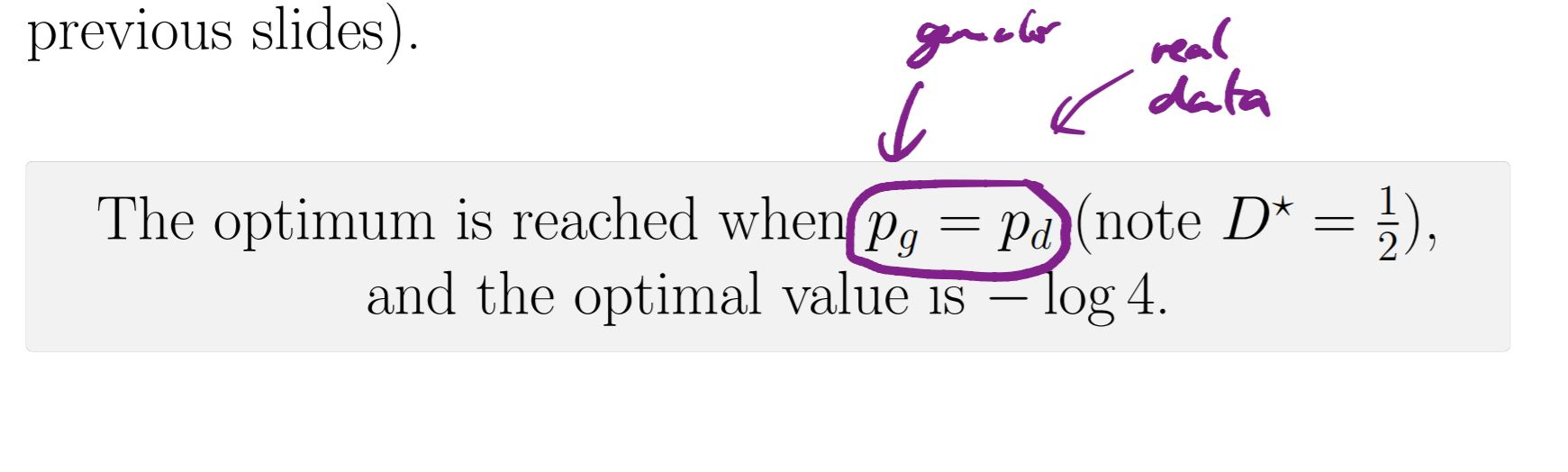### GAN NE and algorithm

Hi,

I was looking at the GANS lecture for the NE definition and was wondering if it is local shouldn't the general condition be followed by " for all theta and phi within a certain neighborhood"? instead of for all theta and phi?Also since the optimum seems to happen when D(x)=1/2 why are we even bothering optimizing it in the algorithm when we can just fix D as a random number generator with equal probability between 1 and 0 since D* seems to be independent of x?Thanks!

I guess D(x)=1/2 only makes sense when you are at the NE, since then adversary G has learned the distribution of data, i.e. p_g = p_d, so the best thing for D to do is make a random unbiased guess. The way I think about it is that D is only there to help G learn, and at the end of training you will obtain a model that can generate realistic images of cats for example.
That's just my intuition, not sure if it's correct.

Hi,

1. for the first part, yes, if the point is local NE, the condition should hold only in a neighborhood of that point.